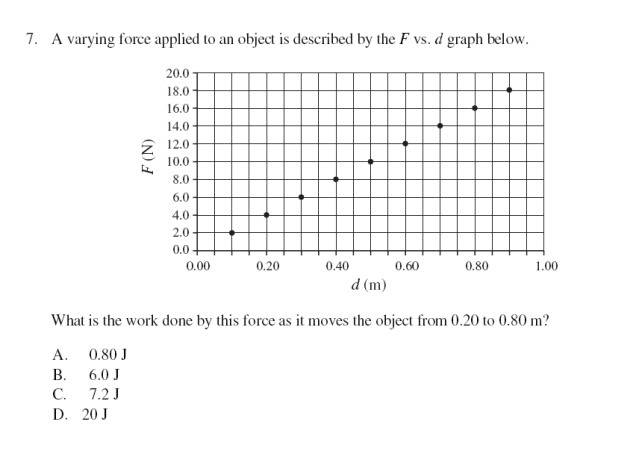# Varying force is applied to an object problem

• brentwoodbc

## Homework StatementI get an answer of 7.2, it says the answer is supposed to be 6.
I do w=fd
w=(16-4)x(.8-.2)
w=12x.6
w=7.2?

thanks.

brentwoodbc said:

## Homework StatementI get an answer of 7.2, it says the answer is supposed to be 6.
I do w=fd
w=(16-4)x(.8-.2)
w=12x.6
w=7.2?

thanks.

The force is varying with distance, not a constant diffrerence of the ending and starting force. You will need to add up all the little F*d contributions to get the total work. You could write an equation for the line, the F(d) line, or you could approximate it as a piece-wise continuous stair-step waveform.

If you have used calculus in your course so far, write the equation for the line and do the integration to sum up the F(d) over the interval. If you haven't use integration for this before, just do the sum for each little interval you see on the graph between the endpoints that they are asking about.